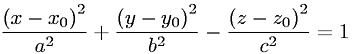Equations > Geometry > Curves and Shapes > Equation of a Hyperboloid of One Sheet

Equation of a Hyperboloid of One SheetLatex Code:

MathML Code:

 $\frac{\left(x-{x}_{0}\right)}^{2}{a}^{2}+\frac{\left(y-{y}_{0}\right)}^{2}{b}^{2}-\frac{\left(z-{z}_{0}\right)}^{2}{c}^{2}=1$

MathType 5.0: